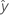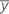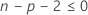# Methods and formulas for Best Subsets Regression

## Computational routine

In best subsets regression, Minitab uses a procedure called the Hamiltonian Walk, which is a method for calculating all possible subsets of predictors, one subset per step. That is, Minitab calculates all 2**m - 1 subsets in 2**m - 1 steps, where m is the number of predictors in the model. Minitab evaluates a different subset regression at each step.

Each subset in the Hamiltonian Walk differs from the preceding subset by the addition or deletion of only one variable. The sweep operator "sweeps" a variable in or out of the regression on each step of the Hamiltonian Walk, and calculates the R2 for each subset.

## Regression equation

For a model with multiple predictors, the equation is:

y = β0 + β1x1 + … + βkxk + ε

The fitted equation is:

In simple linear regression, which includes only one predictor, the model is:

y=ß0+ ß1x1+ε

Using regression estimates b0 for ß0, and b1 for ß1, the fitted equation is:

### Notation

TermDescription
yresponse
xkkth term. Each term can be a single predictor, a polynomial term, or an interaction term.
ßkkth population regression coefficient
εerror term that follows a normal distribution with a mean of 0
bkestimate of kth population regression coefficientfitted response

## R-sq

R2 is also known as the coefficient of determination.

### Notation

TermDescription
yi i th observed response valuemean responsei th fitted response

### Notation

TermDescription
MSMean Square
SSSum of Squares
DFDegrees of Freedom

## PRESS

Assesses your model's predictive ability and is calculated as:

### Notation

TermDescription
nnumber of observations
eiith residual
hi

ith diagonal element of

X (X' X)-1X'

## R-sq (pred)

While the calculations for R2(pred) can produce negative values, Minitab displays zero for these cases.

### Notation

TermDescription
yi i th observed response valuemean response
n number of observations
ei i th residual
hi i th diagonal element of X(X'X)–1X'
X design matrix

## Mallows' Cp

### Notation

TermDescription
SSEpsum of squared errors for the model under consideration
MSEmmean square error for the model with all candidate terms
nnumber of observations
pnumber of terms in the model, including the constant

## S

### Notation

TermDescription
MSEmean square error

## Log-likelihood

For unweighted analyses, Minitab uses the following equation:
For an analysis that has weights for the observations, Minitab uses the following equation:

Observations with weights of 0 are not in the analysis.

### Notation

TermDescription
nthe number of observations
Rthe sum of squares for error for the model
withe weight of the ith observation

## AICc (Akaike's Corrected Information Criterion)

AICc is not calculated when.

### Notation

TermDescription
nthe number of observations
pthe number of coefficients in the model, including the constant

## BIC (Bayesian Information Criterion)

### Notation

TermDescription
pthe number of coefficients in the model, including the constant
nthe number of observations

## Condition number

### Notation

TermDescription
Cthe condition number
λmaximum the maximum eigenvalue from the correlation matrix of the terms in the model, not including the intercept
λminimum the minimum eigenvalue from the correlation matrix of the terms in the model, not including the intercept
By using this site you agree to the use of cookies for analytics and personalized content.  Read our policy